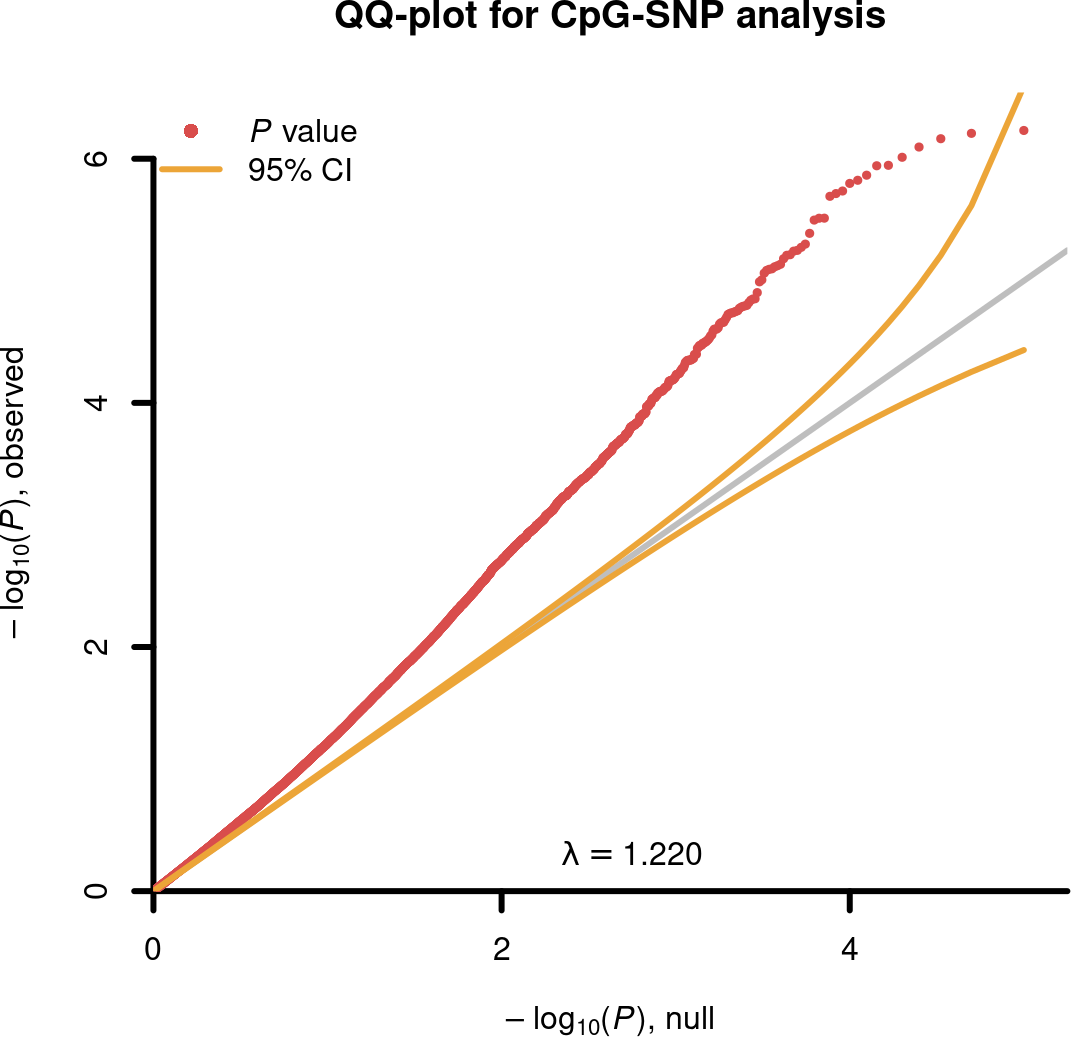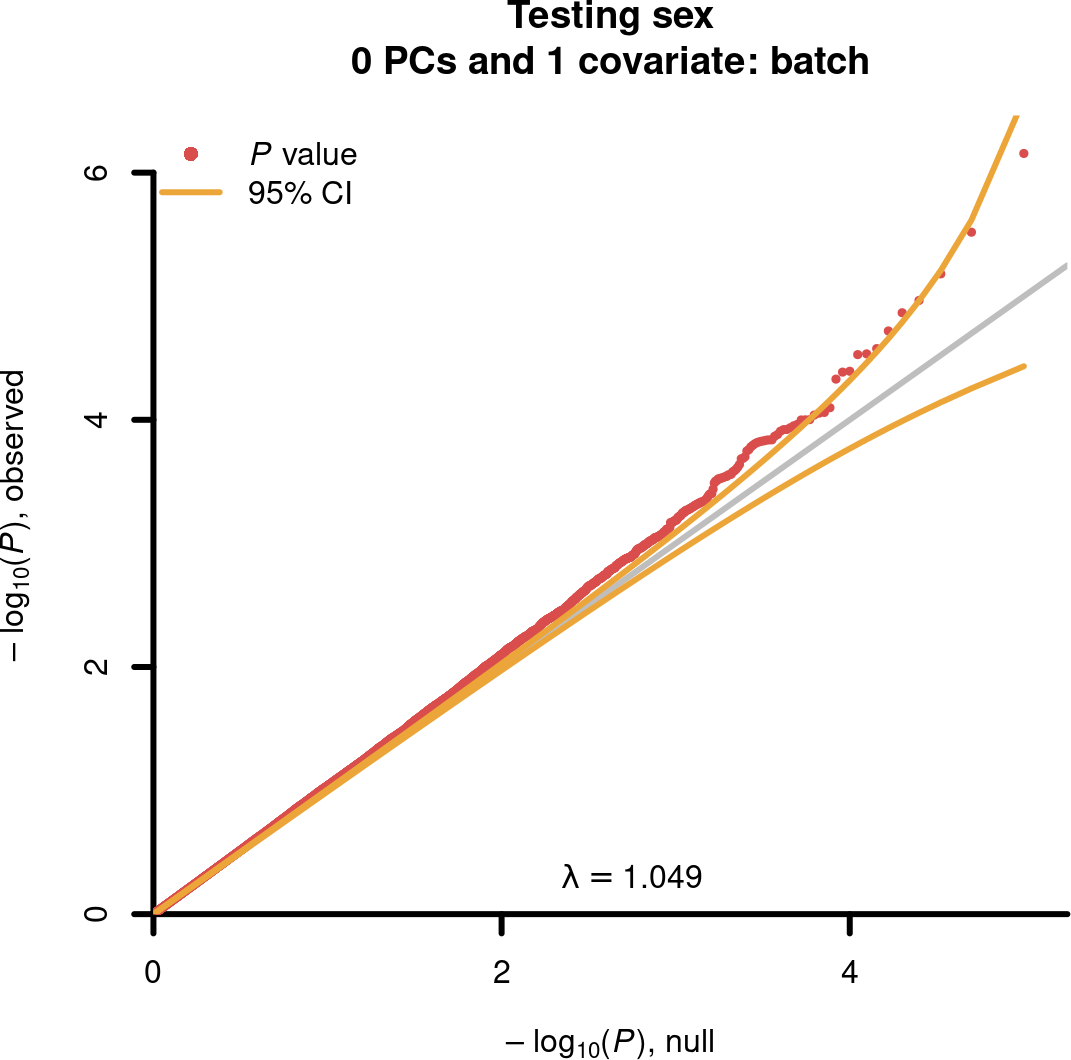# 1 Statistical model for Joint Analysis of Methylation and Genotype Data

Single nucleotide polymorphisms (SNPs) can create and destroy CpGs. As methylation occurs mostly at CpGs, such CpG-SNPs can directly affect methylation measurements.

Recall that enrichment-based methylation methods measure total methylation in a vicinity of a CpG. By creating or destroying a CpG, CpG-SNPs introduce a variation in the total methylation in a vicinity of the CpG which can greatly reduce our power to detect case-control differences.

RaMWAS can account for a possible effect of CpG-SNPs by testing for joint significance of $$\beta_1$$ and $$\beta_2$$ the following model:

$\mu_i = \beta_0 + outcome * \beta_1 + {outcome} * {SNP}_i * \beta_2 + {SNP}_i * \beta_3 + \gamma * { cvrt} + \epsilon$

where

• $$\mu_i$$ – methylation measurement for $$i$$-th CpG.
• $$outcome$$ – phenotype of interest.
• $$SNP_i$$ – the SNP values (0/1/2 or dosages for imputed genotype) at the $$i$$-th CpG.
• $$cvrt$$ – covariates and the principal components.
• $$\epsilon$$ – noise.

# 2 Input data

For CpG-SNPs analysis RaMWAS requires the usual input (see steps 4 and 5) with an additional SNP matrix.

The SNP data must have the same dimensions as the CpG score matrix, i.e. it must be available for the same set of samples and the same set of locations. Data preparation may include finding the closest SNP for every CpG and exclusion of CpGs without any SNPs in vicinity.

## 2.1 Create data matrices for CpG-SNP analysis

To illustrate this type of analysis we produce the following artificial files.

• CpG_locations.* – filematrix with the location of the SNP-CpGs.
It has two columns with integer values – chromosome number and location (chr and position).
• CpG_chromosome_names.txt – file with chromosome names (factor levels) for the integer column chr in the location filematrix.
• Coverage.* – filematrix with the data for all samples and all locations.
Each row has data for a single sample. Row names are sample names.
Each column has data for a single location. Columns match rows of the location filematrix.
• SNPs.* – filematrix with genotype data, matching the coverage matrix.

First, we load the package and set up a working directory. The project directory dr can be set to a more convenient location when running the code.

library(ramwas)

# work in a temporary directory
dr = paste0(tempdir(), "/simulated_matrix_data")
dir.create(dr, showWarnings = FALSE)
cat(dr,"\n")
## /tmp/Rtmpiez5os/simulated_matrix_data

Let the sample data matrix have 200 samples and 100,000 variables.

nsamples = 200
nvariables = 100000

For these 200 samples we generate a data frame with age and sex phenotypes and a batch effect covariate.

covariates = data.frame(
sample = paste0("Sample_",seq_len(nsamples)),
sex = seq_len(nsamples) %% 2,
age = runif(nsamples, min = 20, max = 80),
batch = paste0("batch",(seq_len(nsamples) %% 3))
)
pander(head(covariates))
sample sex age batch
Sample_1 1 71.5 batch1
Sample_2 0 35.8 batch2
Sample_3 1 60.4 batch0
Sample_4 0 64.5 batch1
Sample_5 1 28.4 batch2
Sample_6 0 26.3 batch0

Next, we create the genomic locations for 100,000 variables.

temp = cumsum(sample(20e7 / nvariables, nvariables, replace = TRUE) + 0)
chr      = as.integer(temp %/% 1e7) + 1L
position = as.integer(temp %% 1e7)

locmat = cbind(chr = chr, position = position)
chrnames = paste0("chr", 1:10)
pander(head(locmat))
chr position
1 958
1 1850
1 2916
1 4390
1 5386
1 6104

Now we save locations in a filematrix and create a text file with chromosome names.

fmloc = fm.create.from.matrix(
filenamebase = paste0(dr, "/CpG_locations"),
mat = locmat)
close(fmloc)
writeLines(con = paste0(dr, "/CpG_chromosome_names.txt"), text = chrnames)

Finally, we create methylation and SNP matrices and populate them.

fmm = fm.create(paste0(dr,"/Coverage"), nrow = nsamples, ncol = nvariables)
fms = fm.create(paste0(dr,"/SNPs"), nrow = nsamples, ncol = nvariables,
size = 1, type = "integer")

# Row names of the matrices are set to sample names
rownames(fmm) = as.character(covariates$sample) rownames(fms) = as.character(covariates$sample)

# The matrices are filled, 2000 variables at a time
byrows = 2000
for( i in seq_len(nvariables/byrows) ){ # i=1
ind = (1:byrows) + byrows*(i-1)

snps = rbinom(n = byrows * nsamples, size = 2, prob = 0.2)
dim(snps) = c(nsamples, byrows)
fms[,ind] = snps

slice = double(nsamples*byrows)
dim(slice) = c(nsamples, byrows)
slice[,  1:225] = slice[,  1:225] + covariates$sex / 50 / sd(covariates$sex)
slice[,101:116] = slice[,101:116] + covariates$age / 16 / sd(covariates$age)
slice = slice +
((as.integer(factor(covariates$batch))+i) %% 3) / 200 + snps / 1.5 + runif(nsamples*byrows) / 2 fmm[,ind] = slice } close(fms) close(fmm) # 3 SNP-CpG analysis Let us test for association between CpG scores and and the sex covariate (modeloutcome parameter) correcting for batch effects (modelcovariates parameter). Save top 20 results (toppvthreshold parameter) in a text file. param = ramwasParameters( dircoveragenorm = dr, covariates = covariates, modelcovariates = "batch", modeloutcome = "sex", toppvthreshold = 20, fileSNPs = "SNPs" ) The CpG-SNP analysis: ramwasSNPs(param) The QQ-plot shows better enrichment with significant p-values.For comparison, we also perform the usual MWAS for these CpGs without regard for SNPs. ramwas5MWAS(param) The QQ-plot shows much weaker signal for the standard MWAS.The top finding are saved in the text files Top_tests.txt for both analyses: # Get the directory with testing results toptbl = read.table( paste0(pfull$dirSNPs, "/Top_tests.txt"),
sep = "\t")
pander(head(toptbl,10))
chr position Ftest pvalue qvalue
chr5 2170316 15.5 5.86e-07 0.0156
chr6 6144158 15.4 6.19e-07 0.0156
chr5 2e+06 15.3 6.85e-07 0.0156
chr2 3662023 15.1 8.02e-07 0.0156
chr6 6011776 14.9 9.72e-07 0.0156
chr7 6101550 14.7 1.13e-06 0.0156
chr8 4049811 14.7 1.14e-06 0.0156
chr3 4139987 14.5 1.36e-06 0.0156
chr3 6020915 14.4 1.5e-06 0.0156
chr1 4057972 14.3 1.59e-06 0.0156

Note that CpG-SNP analysis tests for joint significance of $$\beta_1$$ and $$\beta_2$$ and thus uses F-test, while regular MWAS uses t-test.

pfull = parameterPreprocess(param)
paste0(pfull\$dirmwas, "/Top_tests.txt"),
pander(head(toptbl,10))